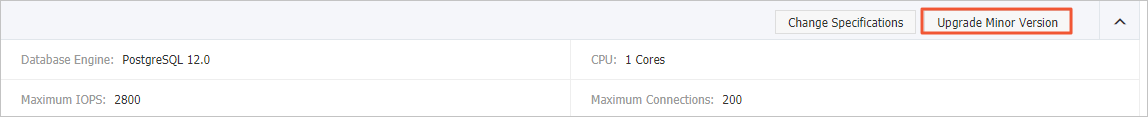This topic describes the use of the HyperLogLog data type supported by the hll plug-in to estimate page views (PV) and unique visitors (UV).

## Prerequisites

The instance runs one of the following PostgreSQL versions:
• PostgreSQL 13
• PostgreSQL 12 (kernel version 20200421 and later)
• PostgreSQL 11 (kernel version 20200402 and later)
Note To view the kernel version, perform the following steps: Log on to the ApsaraDB for RDS console, find the target RDS instance, and navigate to the Basic Information page. Then, in the Configuration Information section, check whether the Upgrade Minor Version button exists. If the button exists, click it to view the kernel version. If the button does not exist, it indicates that you are already using the latest kernel version. For more information, see Upgrade the kernel version of an ApsaraDB RDS for PostgreSQL instance.## Background information

The hll plug-in supports an extendable, set-resembled data type HyperLogLog (hll) to estimate DISTINCT elements under a specified accuracy. For example, you can use 1,280 bytes of hll data to accurately estimate billions of DISTINCT elements. The hll plug-in is suitable for industries that need estimation analysis, such as Internet advertisement analysis to estimate PVs and UVs.

For more information about the detailed algorithm, visit HyperLogLog: the analysis of a near-optimal cardinality estimation algorithm.

## Create an hll plug-in

After you connect to an instance, execute the following statement to create an hll plug-in:

``CREATE EXTENSION hll;``

## Basic operations

• Execute the following statement to create a table that contains hll fields:
``create table agg (id int primary key,userids hll);``
• Execute the following statement to convert INT data to hll_hashval data:
``select 1::hll_hashval;``

## Basic operators

• The hll data type supports the following operators:
• =
• ! =
• <>
• ||
• #

Examples:

``````select hll_add_agg(1::hll_hashval) = hll_add_agg(2::hll_hashval);
• The hll_hashval data type supports the following operators:
• =
• ! =
• <>

Examples:

``````select 1::hll_hashval = 2::hll_hashval;
select 1::hll_hashval <> 2::hll_hashval;``````

## Basic functions

• The hll plug-in supports hash functions such as hll_hash_boolean, hll_hash_smallint, and hll_hash_bigint. Examples:
``````select hll_hash_boolean(true);
select hll_hash_integer(1);``````
• The hll plug-in supports the hll_add_agg function to convert the data type from INT to hll. Example:
``select hll_add_agg(1::hll_hashval);``
• The hll plus-in supports the hll_union function to perform UNION operations on hll data. Example:
``select hll_union(hll_add_agg(1::hll_hashval),hll_add_agg(2::hll_hashval));``
• The hll plus-in supports the hll_set_defaults function to set the accuracy. Example:
``select hll_set_defaults(15,5,-1,1);``
• The hll plug-in supports the hll_print function to display debug information. Example:
``select hll_print(hll_add_agg(1::hll_hashval));``

## Example

``````create table access_date (acc_date date unique, userids hll);
insert into access_date select current_date, hll_add_agg(hll_hash_integer(user_id)) from generate_series(1,10000) t(user_id);
insert into access_date select current_date-1, hll_add_agg(hll_hash_integer(user_id)) from generate_series(5000,20000) t(user_id);
insert into access_date select current_date-2, hll_add_agg(hll_hash_integer(user_id)) from generate_series(9000,40000) t(user_id);
postgres=# select #userids from access_date where acc_date=current_date;
? column?
------------------
9725.85273370708
(1 row)
postgres=# select #userids from access_date where acc_date=current_date-1;
? column?
------------------
14968.6596883279
(1 row)
postgres=# select #userids from access_date where acc_date=current_date-2;
? column?
------------------
29361.5209149911
(1 row)``````